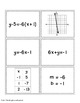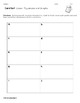# Linear Equations and Graphs Card Sorts and ActivitiesSubject
Resource Type
File Type
PDF (1 MB|15 pages)
\$4.95
Product Description
These fun and challenging activities are a great way for students to practice and learn about linear equations, their graphs and solutions. This product includes a set of 60 cards that will help students make connections between different representations of lines (equations, graphs, slope and y-intercepts and tables of values/solutions).
Activities include three different card games and two card sorting activities.
The first card game, played similar to "Rummy", has students pairing up graphs with equations and tables of values.
The second card game, similar to "Slap Jack", has students connecting graphs to their equations.
The third game is played like concentration where students match up two types of cards.
In the card sorting activities, students look at all of the cards and sort them into matching groups of equations, graphs, tables of values and slope and y-intercept cards or into graphs and equations only. A great discussion activity for small groups of students!

YOU MIGHT ALSO LIKE
Write Linear Equations in Slope Intercept Form Scavenger Hunt Activity
Graphing Lines in Slope Intercept Form Race Game
Linear Equations and Graphs Card Sorts and Activities
Graph Lines from Slope-Intercept Form PowerPoint and GAME
Categories Math Game Lines
Slope Formula Investigation Activity and Notes
Slope Task Cards Common Core Aligned
Solve Linear Equations Game Packet
Total Pages
15 pages